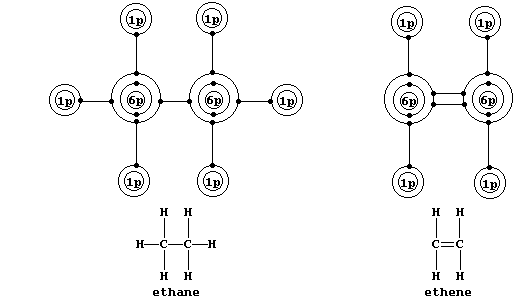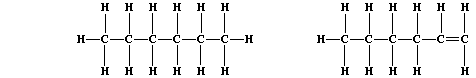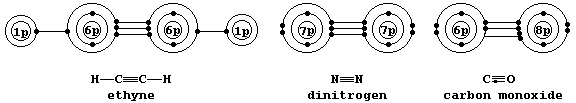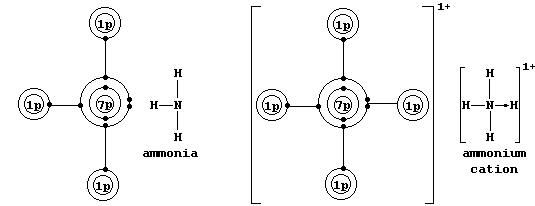```SELECTED PRINCIPLES: INTRODUCTION - LOCALIZED COVALENT BONDING (2)
Multiple bonding will occur between atoms if the resulting molecule or
compound has a lower energy than that achieved by single bonding. *
Localized Double Bonds
Shown below are electron-structure diagrams for ethane and ethene.``````Ethene contains what is (universally) known as a double bond. However,
perhaps contrary to one's initial expectations, these two bonds are not
identical: one of these, known as a s (sigma) bond, consists of two
electrons at one molecular energy level; and the other, known as a p
(pi) bond, consists of two electrons at another molecular energy level.
These equations summarize the partial dissociation of ethene (Eq. 1)
and the complete dissociation of ethene (Eq. 2) and ethane (Eq. 3):
Eq. 1           H2C=CH2 ——————————® H2C-CH2          DH = +264 kJ mol-ą
Eq. 2           H2C=CH2 ——————————® H2C + CH2        DH = +610 kJ mol-ą
Eq. 3           H3C-CH3 ——————————® H3C + CH3        DH = +346 kJ mol-ą
Equations 2 and 3 show that the double bond is stronger than the single
bond by 264 kJ mol-ą; and Equations 1 and 3 show that the p bond is
weaker than the s bond by 82 kJ mol-ą. Despite the increased strength
imparted by double bonding, ethene is more reactive than ethane; e.g.,
in the absence of light, bromine reacts with ethene but not ethane. #
A partial explanation for this apparent anomaly is as follows: both
molecules contain a s bond between the carbons, but bromine reacts with
the (localized) p bond present only in ethene.
*  No attempt will be made here to explain (in detail) the reasons why
some pairs of atoms have little or no tendency to form multiple bonds;
e.g., in contrast to the vast numbers of compounds which contain C=C
bonds, those with Si=Si bonds are very rare indeed.``````#  Demonstrating the differences in chemical reactivity between ethane
and ethene is cumbrous, because both are gases at r.t.p.; the simplest
homologues of the alkanes (CnH2n+2) and alkenes (CnH2n) which are not
either gases or highly volatile liquids, and which can be investigated
safely and conveniently, are hexane and hex-1-ene, respectively.```

```Localized Triple Bonds
Shown below are electron-structure diagrams for ethyne, dinitrogen, and
carbon monoxide.``````Each of these molecules contains what is known as a triple bond; i.e.,
one s bond and two p bonds (p1 and p2), with each bond consisting of
two electrons in different molecular energy levels that encompass the
nuclei of the principal atoms (i.e., C, N, and O). Although each bonded
principal atom contains an octet of electrons, the different manner by
which this is achieved in carbon monoxide merits describing the bonding
in detail. Setting aside each lone-pair, localized about its respective
nucleus, a description of the triple bonding in carbon monoxide is as
follows: one s bond, containing one electron from each atom; one p bond
(p1), containing one electron from each atom; and another p bond (p2),
containing two electrons from the oxygen atom.```
 ` ` ``` Nominal bond energy of each bond / kJ mol-ą``` ``` Total bond energy / kJ mol-ą``` ` ` ` s` ` p1` ` p2` ` S s + p1 + p2` ` Ethyne (HCşCH)` ` 346` ` 264` ` 205` ` 815` ` Dinitrogen (NşN)` ` 167` ` 251` ` 528` ` 946` ` Carbon monoxide (CşO) ` ` 360` ` 385` ` 324` ` 1069`
```The above Table reveals that each of these triple bonds is very strong
but that the strength of each component varies within and between the
three molecules. Their (relative) difficulty of reduction by dihydrogen
is NşN >> CşO > HCşCH; an order that parallels the energy of the p2 bond
(NşN >> CşO > HCşHC), but not the total bond energy (CşO > NşN > HCşCH).
This correlation may just be coincidental, however; certainly, other
bonding parameters, including molecular energy levels and polarization,
are known to influence the rate of reaction. #
*  A covalent bond in which one of the atoms donates both electrons is
called either a coordinate or a dative bond; such bonds are often, but
not always, represented by an arrow showing the direction of donation.
Coordinate bonding is very common (particularly so in compounds of the
transition elements); one example is an ammonium ion, in which nitrogen
uses its lone-pair to form a coordinate bond with a hydrogen ion.``````#  Here, for example, it is noteworthy that dinitrogen is non-polar,
whereas carbon monoxide and ethyne are both polar molecules (with an
unequal distribution of 'electron density' about the nuclei).
Dr. R. Peters                   Next                     Contents' List```